# ISEE Middle Level Math : How to find the decimal equivalent of a fraction

## Example Questions

### Example Question #51 : Fractions

Which is equivalent to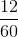?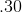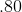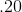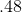Explanation:

Divide: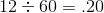Answer:### Example Question #52 : Fractions

Which is equivalent to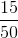?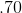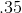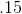Explanation:

Divide: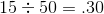Answer:### Example Question #53 : Fractions

Which is equivalent to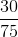?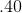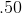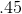Explanation:

Divide: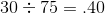Answer:### Example Question #54 : Fractions

What is the equivalent of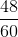?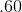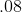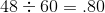Explanation:

Divide:Answer:### Example Question #55 : Fractions

What is the equivalent of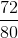?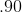9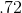Explanation:

Divide: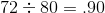Answer:### Example Question #56 : Fractions

What is the equivalent of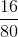?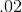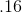Explanation:

Divide: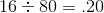Answer:### Example Question #57 : Fractions

Find the equivalent of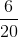.Explanation:

To find the equivalent divide: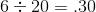Answer:The equivalent is### Example Question #58 : Fractions

Find the equivalent of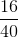.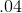Explanation:

To find the equivalent divide: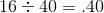Answer:The equivalent is### Example Question #59 : Fractions

Find the decimal equivalent of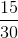.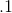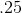Explanation:

To find the decimal equivalent, divide the numerator by the denominator: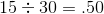Alternatively, you might notice that 15 is half of 30 and skip the actual division.

Answer: The decimal equivalent is.

### Example Question #60 : Fractions

Find the decimal equivalent of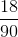.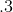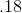Explanation:

To find the decimal equivalent, divide the numerator by the denominator: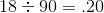Answer: The decimal equivalent is.

### All ISEE Middle Level Math Resources